# Surface Area Calculator

Use this surface area calculator to easily calculate the surface area of common 3-dimensional bodies like a cube, rectangular box, cylinder, sphere, cone, and triangular prism. Total surface area formulas and explanation below.

Share calculator:

Embed this tool:
get code

## How to calculate the surface area of a body?

Depending on the type of body, there are different formulas and different required information you need to calculate surface area (a.k.a. total surface area). Below are the formulas for calculating surface area of the most common body types.

In all surface area calculations, make sure that all lengths are measured in the same unit, e.g. inches, feet, mm, cm. The result from our surface area calculator will always be a square of the same unit: square feet, square inches, square meters, square cm, square mm. etc.

## Surface area of a cube

The surface area formula for a cube is 6 x side2, as seen in the figure below: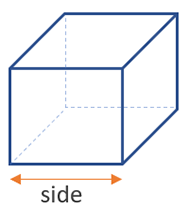This calculation requires only one measurement, due to the symmetricity of the cube. side2 is the surface of one of the sides, and since the cube has 6 equal sides, multiplying by 6 gives us the total cube surface area.

## Surface area of a box

The surface area formula for a rectangular box is 2 x (height x width + width x length + height x length), as seen in the figure below: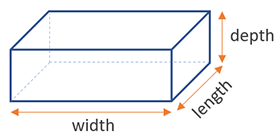Since a rectangular box or tank has opposite sides which are equal, we calculate each unique side's area, then add them up together, and finally multiply by two to find the total surface area.

## Surface area of a cylinder

The surface area formula for a cylinder is π x diameter x (diameter / 2 + height), where (diameter / 2) is the radius of the base (d = 2 x r), so another way to write it is π x radius x 2 x (radius + height). Visual in the figure below: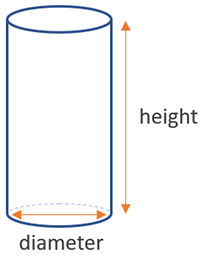The cylinder surface area is the height times the perimeter of the circle base, plus the areas of the two bases, all added together.

## Surface area of a sphere

The surface area formula for a sphere is 4 x π x (diameter / 2)2, where (diameter / 2) is the radius of the sphere (d = 2 x r), so another way to write it is 4 x π x radius2. Visual on the figure below: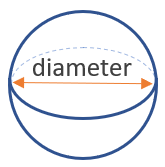A sphere's surface area can be calculated just by knowing its diameter, or radius (diameter = 2 x radius). π is, of course, the well-known mathematical constant, about equal to 3.14159. To find the SA simply multiply 4 times 3.14159 times the radius square.

## Surface area of a cone

The surface area formula for a cone, given its diameter (or radius) and height is π x (diameter / 2)2 + π x (diameter / 2) x √ ((diameter / 2)2 + (height2)), where (diameter / 2) is the radius of the base (d = 2 x r), so another way to write it is π x radius2 + π x radius x √ (radius2 + (height2)), as seen in the figure below: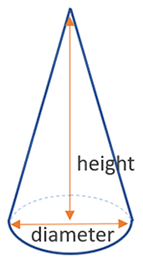The surface area of a cone is one of the most complicated and it is where the need for a calculator becomes more apparent. You need just two measurements: the diameter of the base and it's height, but the calculus is more involved than most of the other simple bodies.

## Surface area of a triangular prism

The surface area formula for a triangular prism is 2 * (height x base / 2) + length x width1 + length x width2 + length x base, as seen in the figure below: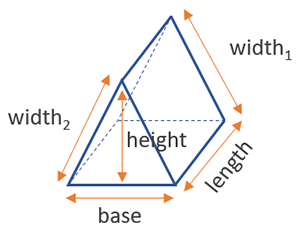A triangular prism is a stack of triangles, so the usually triangle solving rules apply when calculating the area of the bases.

#### Cite this calculator & page

If you'd like to cite this online calculator resource and information as provided on the page, you can use the following citation:
Georgiev G.Z., "Surface Area Calculator", [online] Available at: https://www.gigacalculator.com/calculators/surface-area-calculator.php URL [Accessed Date: 06 Jun, 2023].